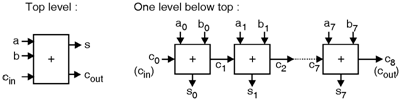Home > VHDL > Arithmetic Circuits > Carry ripple adder

Figure below shows the 8-bit unsigned carry ripple adder. The detailed diagram shows the inputs and outputs signals of the circuit. The a and b are the input vectors to be added, cin is the carry-in bit, s is the sum vector, and cout is the carry-out bit.```LIBRARY ieee;
USE ieee.std_logic_1164.all;
GENERIC (length : INTEGER := 8);
PORT ( a, b: IN STD_LOGIC_VECTOR (length-1 DOWNTO 0);
cin: IN STD_LOGIC;
s: OUT STD_LOGIC_VECTOR (length-1 DOWNTO 0);
cout: OUT STD_LOGIC);
BEGIN
PROCESS (a, b, cin)
VARIABLE carry : STD_LOGIC_VECTOR (length DOWNTO 0);
BEGIN
carry(0) := cin;
FOR i IN 0 TO length-1 LOOP
s(i) <= a(i) XOR b(i) XOR carry(i);
carry(i+1) := (a(i) AND b(i)) OR (a(i) AND
carry(i)) OR (b(i) AND carry(i));
END LOOP;
cout <= carry(length);
END PROCESS;